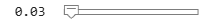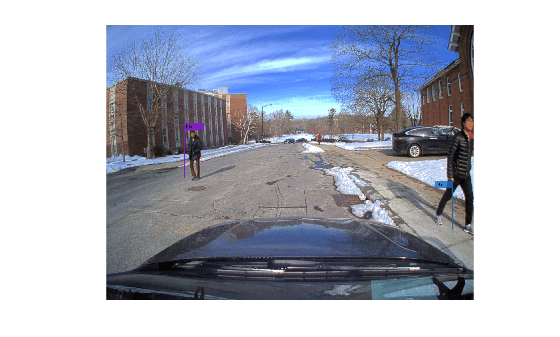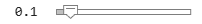# Implement Simple Online and Realtime Tracking

This example shows how to implement the Simple Online and Realtime (SORT) object tracking algorithm  using the Sensor Fusion and Tracking Toolbox™ and the Computer Vision Toolbox™. The example also shows how to evaluate SORT with the CLEAR MOT metrics.

```datasetname="PedestrianTracking"; videoURL = "https://ssd.mathworks.com/supportfiles/vision/data/PedestrianTrackingVideo.avi"; if ~exist("PedestrianTrackingVideo.avi","file") disp("Downloading Pedestrian Tracking Video (35 MB)") websave("PedestrianTrackingVideo.avi",videoURL); end```
```Downloading Pedestrian Tracking Video (35 MB) ```

Refer to the Import Camera-Based Datasets in MOT Challenge Format for Object Tracking (Sensor Fusion and Tracking Toolbox) example to learn how to import the ground truth and detection data into appropriate Sensor Fusion and Tracking Toolbox™ formats. You use the same pedestrian tracking dataset in this example. This example provides two sets of detections for the video. The `PedestrianTrackingACFDetections` MAT-file contains detections generated from a people detector using aggregate channel features (ACF). See the `peopleDetectorACF` function for more details. The `PedestrianTrackingYOLODetections` MAT-file contains detections generated from a YOLO v4 object detector using CSP-DarkNet-53 network and trained on the COCO dataset. See the `yolov4ObjectDetector` object for more details. Both detections sets are saved in the `objectDetection` (Sensor Fusion and Tracking Toolbox) format. Use the ACF detections first.

`load("PedestrianTrackingACFDetections.mat","detections");`

### Define Tracker Components for SORT

The SORT algorithm is a multi-object tracker with the following characteristics:

• Estimation Filter: A Kalman filter with a constant velocity motion model.

• Association Cost: Intersection over union of bounding boxes of detections relative to bounding boxes of predicted tracks.

• Association Type: Global Nearest Neighbor using the Hungarian algorithm.

• Track Maintenance: Initialization and deletion of tracks based on the track history logic. Refer to the Introduction to Track Logic (Sensor Fusion and Tracking Toolbox) example for more details.

#### Define Kalman Filter

The detection measurement is a 2D bounding box:

`$\mathit{Z}=\left[\mathit{x},\mathit{y},\mathit{w},\mathit{h}\right]$`

where $\mathit{x}$ and $\mathit{y}$ are the coordinates of the top-left corner of the bounding box in pixels, and $\mathit{w}$ and $\mathit{h}$ are the width and height of the bounding box in pixels, respectively.

The state of the estimated bounding box follows the definition below:

`$\mathit{X}=\left[\mathit{u},\mathit{v},\mathit{s},\mathit{r},\stackrel{˙}{\mathit{u}},\stackrel{˙}{\mathit{v}},\stackrel{˙}{\mathit{s}},\stackrel{˙}{\text{\hspace{0.17em}}\mathit{r}}\right]$`

where $\mathit{u}$ and $\mathit{v}$ are the coordinates of the center of the bounding box, $\mathit{s}$ is the scale (or area) of the bounding box, and $\mathit{r}$ is the width-to-height ratio of the bounding box. The last four elements are the time rate of change of the first four elements, respectively. Unlike in , the time rate of change of the aspect ratio is included in the state in this example.

The equations to convert measurement to state are therefore given by:

`$\begin{array}{l}\mathit{u}=\mathit{x}+\frac{\mathit{w}}{2}\\ \mathit{v}=\mathit{y}+\frac{\mathit{h}}{2}\\ \mathit{s}=\mathit{w}\text{\hspace{0.17em}}\mathit{h}\\ \mathit{r}=\frac{\mathit{w}}{\mathit{h}}\end{array}$`

Note that the equations are nonlinear. The conversion can be done as a pre-processing step outside of the filter. As a result you can use a linear Kalman filter with a `trackingKF` (Sensor Fusion and Tracking Toolbox) object. Alternatively, the conversion can be done in the Kalman filter measurement function, which requires an extended Kalman filter to handle the nonlinearity. This example uses the first approach, which is also adopted in . To set up a measurement function for the second approach, use the `helperBBMeasurementFcn` function provided with this example.

Assume the detection noise is zero-mean Gaussian, with a covariance $\mathit{R}$ that corresponds to a standard deviation of $1$ for the center position and the aspect-ratio. It also has a standard deviation of$\sqrt{10}$ pixels for the scale.

`$\mathit{R}=\left[\begin{array}{cccc}1& 0& 0& 0\\ 0& 1& 0& 0\\ 0& 0& 10& 0\\ 0& 0& 0& 1\end{array}\right]$`

Use the `helperConvertBoundingBox` function to convert all the detections to the state convention and set the measurement noise covariance.

```R = diag([1, 1, 10, 1]); convertedDets = helperConvertBoundingBox(detections,R);```

The state transition from times ${\mathit{t}}_{\mathit{k}\text{\hspace{0.17em}}}$to ${\mathit{t}}_{\mathit{k}+1}={\mathit{t}}_{\mathit{k}}+{\delta }_{\mathit{t}}$ follows a constant velocity model given by:

`${\mathit{X}}_{\mathit{k}+1}=\mathit{A}\text{\hspace{0.17em}}{\mathit{X}}_{\mathit{k}}=\left[\begin{array}{cc}{\mathit{I}}_{4}& {\delta }_{\mathit{t}}×{\text{\hspace{0.17em}}\mathit{I}}_{4}\\ {0}_{4\text{\hspace{0.17em}}}& {\mathit{I}}_{4}\end{array}\right]{\mathit{X}}_{\mathit{k}}\text{\hspace{0.17em}}$`

In this example, the video has 1 frame per second and therefore ${\delta }_{\mathit{t}}=1$. Adjust the value accordingly if you use a different video.

Initialize the velocity state with zero velocity and a large standard deviation to represent high motion uncertainty.

The constant velocity model is a crude approximation and does not accurately describe the actual motion of the pedestrians in the video, nor the variations of the area and aspect-ratio states. As shown in the results below, a larger process noise for the $\stackrel{˙}{\mathit{u}},\stackrel{˙}{\mathit{v}},\stackrel{˙}{\mathit{s}}$ state elements produces desirable results for this application with the current choice of measurement noise.

The `helperInitcvbbkf` function constructs the Kalman filter from an initial detection. You can modify this function for your application.

```function filter = helperInitcvbbkf(detection) % Initialize a linear Constant-Velocity Kalman filter for Bounding Box tracking. % Detection must have a measurement following the [u, v, s, r] format measurement = detection.Measurement; % Initialize state with null velocity X0 = [measurement(1:4)' ; zeros(4,1)]; % Initialize state covariance with high variance on velocity states P0 = diag([1 1 10 10 1e4 1e4 1e4 1e2]); % Add some process noise to capture unknown acceleration Q = diag([1 1 1 1 10 10 10 1]); dt = 1; A = [eye(4), dt*eye(4); zeros(4), eye(4)]; H = [eye(4), zeros(4)]; % Construct the filter filter = trackingKF(State = X0,... StateCovariance = P0,... ProcessNoise = Q, ... MotionModel = "custom",... StateTransitionModel = A,... MeasurementModel = H); end ```

#### Define Association Cost Function and Association Threshold

In SORT, association between bounding box detections and current tracks requires the calculation of association cost between each detection and each current track. Also, a lower cost must indicate that the detection is more likely to originate from the paired track. Use the `bboxOverlapRatio` function from Computer Vision Toolbox™ to calculate the intersection over union similarity for each detection and track pair. You must convert the detection measurements and track states back to the initial bounding box format before using `bboxOverlapRatio`.

```function iou = similarityIoU(tracks, detections) % Calculate the Intersection over Union similarity between tracks and % detections states = [tracks.State]; bbstate = helperBBMeasurementFcn(states); % Convert states to [x, y, w, h] for bboxOverlapRatio bbmeas = vertcat(detections.Measurement); bbmeas = helperBBMeasurementFcn(bbmeas')'; iou = bboxOverlapRatio(bbstate', bbmeas); % Bounding boxes must be concatenated vertically end ```

The overlap ratio is a measure of similarity and a higher value indicates a stronger match. Therefore, you use the negative of the similarity as the cost value. The `helperSORTCost` function predicts all current tracks maintained by the tracker and formulates the cost matrix for all detection-track pairs.

```function costMatrix = helperSORTCost(tracker, dets) D = numel(dets); T = tracker.NumTracks; % Return early if no detections or no tracks if D*T == 0 costMatrix = zeros(T,D); return end time = dets(1).Time; tracks = predictTracksToTime(tracker, "all",time); costMatrix = -similarityIoU(tracks, dets); end ```

Like in most multi-object tracking algorithms, setting a threshold for the association of detections to tracks is beneficial in SORT. When the association cost exceeds this threshold, the assignment is forbidden. You formulate the threshold as the minimum similarity `IoUmin.` This parameter of the SORT algorithm should be tuned for each application. For the video used in this example, a minimum similarity value of 0.05 gives good results due to the low density of pedestrian and the low framerate.

`IoUmin =0.05;`

Set the `AssignmentThreshold` property of the tracker to the negative of the minimum similarity in the following section.

#### Use Global Nearest Neighbor Association

SORT relies on a one-to-one association between detections and tracks by finding the minimal cost of association. This is also known as global nearest neighbor (GNN) in the field of multi-object tracking. Therefore, you can use the `trackerGNN` (Sensor Fusion and Tracking Toolbox) System Object™ to implement SORT. When creating the tracker, specify the tracking filter initialization function as `helperInitcvbbkf` and set the `HasCostMatrixInput` property to `true` to use the custom `helperSortCost` function instead of the default cost calculation.

```tracker = trackerGNN(FilterInitializationFcn=@helperInitcvbbkf,... HasCostMatrixInput=true,... AssignmentThreshold= -IoUmin);```

#### Define Track Maintenance

Objects can leave the video frame or become occluded for brief or long periods. You need to define the maximum number of frames without assigned detections, ${\mathit{T}}_{\mathrm{Lost}}$ , before deleting a track. The tracker parameter ${\mathit{T}}_{\mathrm{Lost}}$ can be tuned for each application and a value of 3 shows good results for this video. Additionally, SORT requires an object to be detected in two consecutive frames before confirming a track. You set the `ConfirmationThreshold` property of the tracker accordingly.

```TLost =3; % Number of consecutive missed frames to delete a track tracker.ConfirmationThreshold=[2 2]; tracker.DeletionThreshold=[TLost TLost];```

### Run SORT with ACF Detections

Run SORT on the video and detections. Filter out ACF detections with a score lower than 15 to improve the tracking performance. You can tune the score threshold for specific scenarios. Log the tracks at each timestep for offline evaluation.

```detectionScoreThreshold = 15; % Initialize track log acfSORTTrackLog = objectTrack.empty; reader = VideoReader(datasetname+"Video.avi"); for i=1:reader.NumFrames % Parse detections set to retrieve detections on the ith frame curFrameDetections = convertedDets{i}; attributes = [curFrameDetections.ObjectAttributes]; scores = [attributes.Score]; highScoreDetections = curFrameDetections(scores > detectionScoreThreshold); % Calculate association cost matrix iouCost = helperSORTCost(tracker,highScoreDetections ); % Update tracker tracks = tracker(highScoreDetections, reader.CurrentTime, iouCost); % Advance reader frame = readFrame(reader); frame = helperAnnotateTrack(tracks, frame); % Uncomment the line below to show detection % frame = helperAnnotateConvertedDetection(highScoreDetections, frame); imshow(frame); % Log tracks for evaluation acfSORTTrackLog = [acfSORTTrackLog ; tracks]; %#ok<AGROW> end```By the end of the video, a pedestrian is tracked with a `trackID` of 45. The sequence contains exactly 16 distinct pedestrians. Apparently, the tracker has confirmed new tracks for the same true object several times as well as possibly confirmed false positive tracks.

SORT can struggle to initiate for tracking fast moving objects because it initializes a tentative track in the first frame with zero velocity and the detection of the same object in the next frame may not overlap with the prediction. This challenge is further accentuated in videos with low framerate like the video in this example. For instance, track 5 is not confirmed until visible for multiple frames.

Notice that pedestrians who leave the field of view of the camera or are occluded by another person for a few frames are lost by the tracker. This result is a combination of using the constant velocity model to predict the position of the track and using the IoU association cost, which cannot associate a predicted track to a new detection if the positions are too far.

The quality of the detections also has noticeable impacts on tracking results. For example, the ACF detections of the tree at the end of the street are associated to track 3.

In the next section, you evaluate SORT with the YOLOv4 detections.

### Run SORT with YOLOv4 Detections

In this section you run SORT with the detections obtained from the YOLOv4 detector. The `helperRunSORT` function repeats the simulation loop from the previous section. The range of scores for YOLOv4 is much higher and the detection quality is sufficiently good such that you do not need to filter out low score detections.

```% Load and convert YOLOv4 detections load("PedestrianTrackingYOLODetections.mat","detections"); convertedDets = helperConvertBoundingBox(detections, R); detectionScoreThreshold = -1; showAnimation = true; yoloSORTTrackLog = helperRunSORT(tracker, convertedDets, detectionScoreThreshold, showAnimation);```The YOLOv4-SORT combination created a total of 24 tracks on the video, indicating that fewer track fragmentations occurred as compared to the ACF detections. From the results, track fragmentations and ID switches are still noticeable.

More recent tracking algorithms, such as DeepSORT, modify the association cost to include appearance features in addition to IoU. These algorithms show great improvements in accuracy and are able to keep tracks over longer occlusions thanks to re-identification networks.

### Evaluate SORT with the CLEAR MOT Metrics

The CLEAR multi-object tracking metrics provide a standard set of tracking metrics to evaluate the quality of tracking algorithm . These metrics are popular for video-based tracking applications. Use the `trackCLEARMetrics` (Sensor Fusion and Tracking Toolbox) object to evaluate the CLEAR metrics for the two SORT runs.

The CLEAR metrics requires a similarity method to match track and true object pairs in each frame. In this example, you use the `IoU2d` similarity method and set the `SimilarityThreshold` property to 0.1. This means that a track can only be considered a true positive match with a truth object if their bounding boxes overlap by at least 10%. The metric results can vary depending on the choice of this threshold.

```threshold =0.1; tcm = trackCLEARMetrics(SimilarityMethod ="IoU2d", SimilarityThreshold = threshold);```

The first step is to convert the `objectTrack` format to the `trackCLEARMetrics` input format specific to the `IoU2d` similarity method. Convert the two logs of tracks obtained previously.

```acfTrackedObjects = repmat(struct("Time",0,"TrackID",1,"BoundingBox", [0 0 0 0]),size(acfSORTTrackLog)); for i=1:numel(acfTrackedObjects) acfTrackedObjects(i).Time = acfSORTTrackLog(i).UpdateTime; acfTrackedObjects(i).TrackID = acfSORTTrackLog(i).TrackID; acfTrackedObjects(i).BoundingBox(:) = helperBBMeasurementFcn(acfSORTTrackLog(i).State(1:4)); end yoloTrackedObjects = repmat(struct("Time",0,"TrackID",1,"BoundingBox", [0 0 0 0]),size(yoloSORTTrackLog)); for i=1:numel(yoloTrackedObjects) yoloTrackedObjects(i).Time = yoloSORTTrackLog(i).UpdateTime; yoloTrackedObjects(i).TrackID = yoloSORTTrackLog(i).TrackID; yoloTrackedObjects(i).BoundingBox(:) = helperBBMeasurementFcn(yoloSORTTrackLog(i).State(1:4)); end```

The `PedestrianTrackingGroundTruth` MAT-file contains the log of truth objects formatted as an array of structures. Each structure contains the following fields: `TruthID`, `Time`, and `BoundingBox`. After loading the ground truth, call the `evaluate` object function to obtain the metrics as a table.

```load("PedestrianTrackingGroundTruth.mat","truths"); acfSORTresults = evaluate(tcm, acfTrackedObjects, truths); yoloSORTresults = evaluate(tcm, yoloTrackedObjects, truths);```

Concatenate the two tables and add a column with the name of each tracker and object detector.

```allResults = [table("ACF+SORT",VariableNames = "Tracker") , acfSORTresults ; ... table("YOLOv4+SORT",VariableNames = "Tracker"), yoloSORTresults]; disp(allResults);```
``` Tracker MOTA (%) MOTP (%) Mostly Tracked (%) Partially Tracked (%) Mostly Lost (%) False Positive False Negative Recall (%) Precision (%) False Track Rate ID Switches Fragmentations _____________ ________ ________ __________________ _____________________ _______________ ______________ ______________ __________ _____________ ________________ ___________ ______________ "ACF+SORT" 64.043 66.943 57.143 35.714 7.1429 15 215 66.821 96.652 0.088757 3 15 "YOLOv4+SORT" 82.099 90.48 78.571 14.286 7.1429 21 94 85.494 96.348 0.12426 1 9 ```

The two main summary metrics are Multi-Object Tracking Accuracy (MOTA) and Multi-Object Tracking Precision (MOTP). MOTA is a good indicator of the data association quality while MOTP indicates the similarity of each track bounding boxes with their matched true bounding boxes. The metrics confirm that the YOLOv4 and SORT combination tracks better than the ACF and SORT combination. It scores roughly 20 percent higher for both MOTA and MOTP.

ID switches and fragmentations are two other metrics that provide good insights on a tracker's ability to track each pedestrian with a unique track ID. Fragmentations can occur when a true object is obstructed and the tracker cannot maintain the track continuously over several frames. ID switches can occur when true objects trajectories are crossing and their assigned track IDs switch afterwards.

Refer to the `trackCLEARMetrics` (Sensor Fusion and Tracking Toolbox) page for additional information about all the CLEAR metrics quantities and their significance.

### Conclusion

In this example you learned how to implement SORT. Also, you evaluated this tracking algorithm on a pedestrian tracking video. You discovered that the overall tracking performance depends strongly on the quality of the detections. You can reuse this example with your own video and detections. Furthermore, you can use the Import Camera-Based Datasets in MOT Challenge Format for Object Tracking (Sensor Fusion and Tracking Toolbox) example to import videos and detections from the MOT Challenge .

### Reference

 Bewley, Alex, Zongyuan Ge, Lionel Ott, Fabio Ramos, and Ben Upcroft. "Simple online and realtime tracking." In 2016 IEEE international conference on image processing (ICIP), pp. 3464-3468. IEEE, 2016.

 Bernardin, Keni, and Rainer Stiefelhagen. "Evaluating multiple object tracking performance: the clear mot metrics." EURASIP Journal on Image and Video Processing 2008 (2008): 1-10.